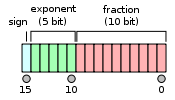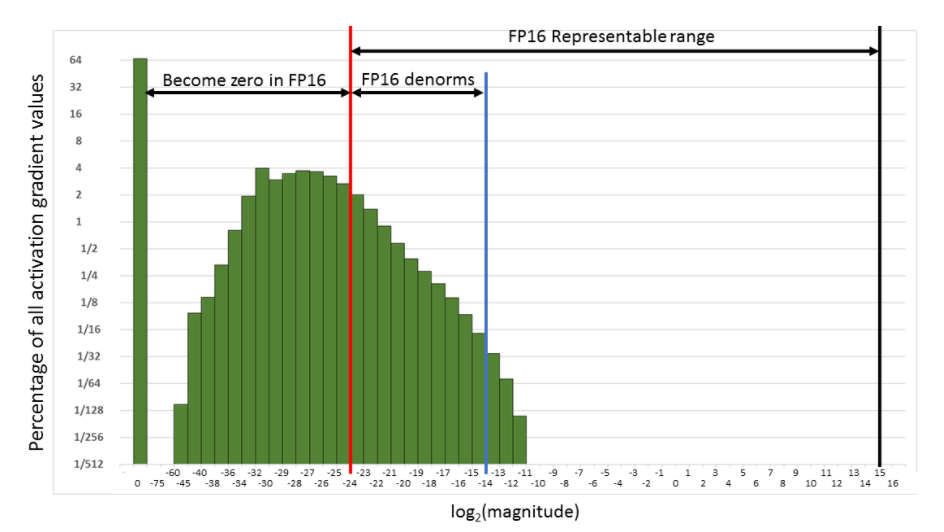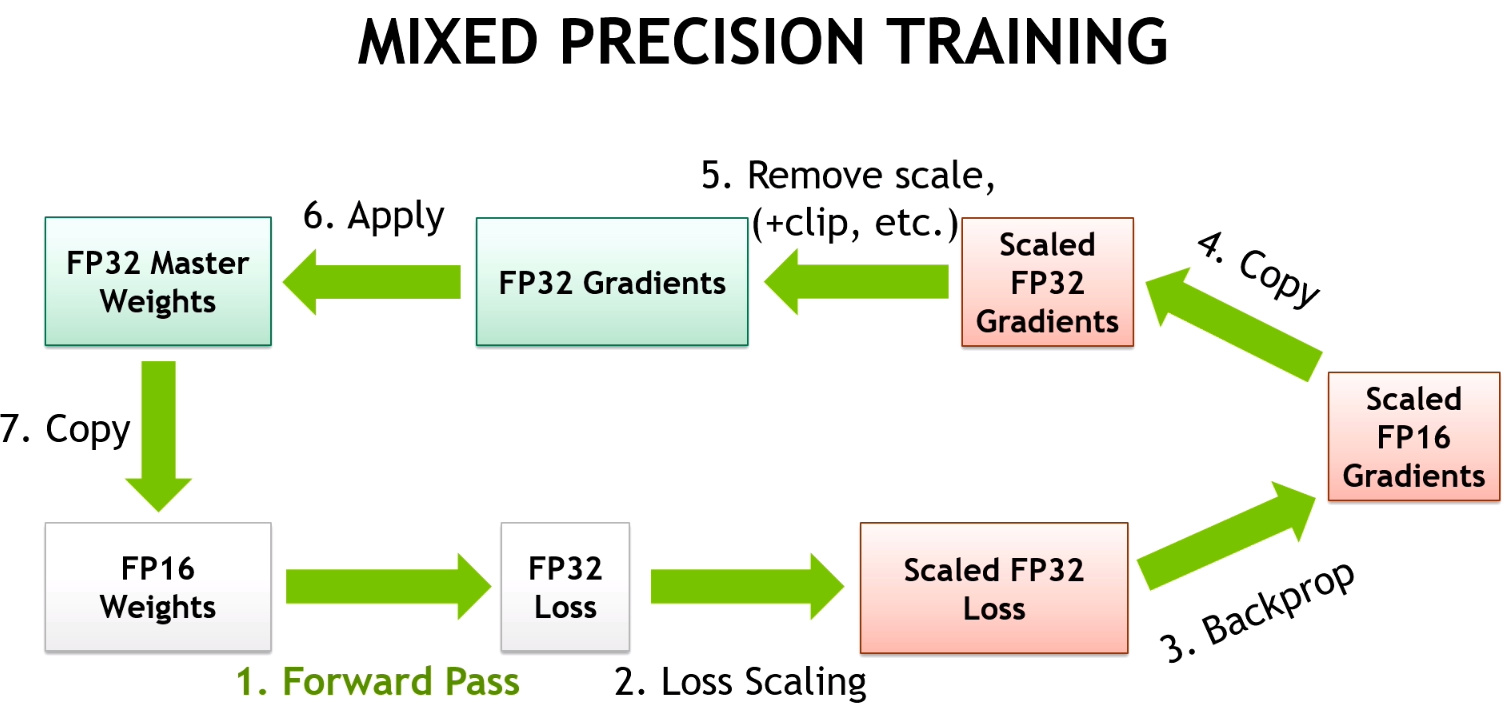Callback and utility functions to allow mixed precision training



## A little bit of theory

A very nice and clear introduction to mixed precision training is this video from NVIDIA.

### What's half precision?

In neural nets, all the computations are usually done in single precision, which means all the floats in all the arrays that represent inputs, activations, weights... are 32-bit floats (FP32 in the rest of this post). An idea to reduce memory usage (and avoid those annoying cuda errors) has been to try and do the same thing in half-precision, which means using 16-bits floats (or FP16 in the rest of this post). By definition, they take half the space in RAM, and in theory could allow you to double the size of your model and double your batch size.

Another very nice feature is that NVIDIA developed its latest GPUs (the Volta generation) to take fully advantage of half-precision tensors. Basically, if you give half-precision tensors to those, they'll stack them so that each core can do more operations at the same time, and theoretically gives an 8x speed-up (sadly, just in theory).

So training at half precision is better for your memory usage, way faster if you have a Volta GPU (still a tiny bit faster if you don't since the computations are easiest). How do we do it? Super easily in pytorch, we just have to put .half() everywhere: on the inputs of our model and all the parameters. Problem is that you usually won't see the same accuracy in the end (so it happens sometimes) because half-precision is... well... not as precise ;).

### Problems with half-precision:

To understand the problems with half precision, let's look briefly at what an FP16 looks like (more information here).The sign bit gives us +1 or -1, then we have 5 bits to code an exponent between -14 and 15, while the fraction part has the remaining 10 bits. Compared to FP32, we have a smaller range of possible values (2e-14 to 2e15 roughly, compared to 2e-126 to 2e127 for FP32) but also a smaller offset.

For instance, between 1 and 2, the FP16 format only represents the number 1, 1+2e-10, 1+2*2e-10... which means that 1 + 0.0001 = 1 in half precision. That's what will cause a certain numbers of problems, specifically three that can occur and mess up your training.

1. The weight update is imprecise: inside your optimizer, you basically do w = w - lr w.grad for each weight of your network. The problem in performing this operation in half precision is that very often, w.grad is several orders of magnitude below w, and the learning rate is also small. The situation where w=1 and lrw.grad is 0.0001 (or lower) is therefore very common, but the update doesn't do anything in those cases.
3. Your activations or loss can overflow. The opposite problem from the gradients: it's easier to hit nan (or infinity) in FP16 precision, and your training might more easily diverge.

### The solution: mixed precision training

To address those three problems, we don't fully train in FP16 precision. As the name mixed training implies, some of the operations will be done in FP16, others in FP32. This is mainly to take care of the first problem listed above. For the next two there are additional tricks.

The main idea is that we want to do the forward pass and the gradient computation in half precision (to go fast) but the update in single precision (to be more precise). It's okay if w and grad are both half floats, but when we do the operation w = w - lr * grad, we need to compute it in FP32. That way our 1 + 0.0001 is going to be 1.0001.

This is why we keep a copy of the weights in FP32 (called master model). Then, our training loop will look like:

1. compute the output with the FP16 model, then the loss
2. back-propagate the gradients in half-precision.
3. copy the gradients in FP32 precision
4. do the update on the master model (in FP32 precision)
5. copy the master model in the FP16 model.

Note that we lose precision during step 5, and that the 1.0001 in one of the weights will go back to 1. But if the next update corresponds to add 0.0001 again, since the optimizer step is done on the master model, the 1.0001 will become 1.0002 and if we eventually go like this up to 1.0005, the FP16 model will be able to tell the difference.

That takes care of problem 1. For the second problem, we use something called gradient scaling: to avoid the gradients getting zeroed by the FP16 precision, we multiply the loss by a scale factor (scale=512 for instance). That way we can push the gradients to the right in the next figure, and have them not become zero.Of course we don't want those 512-scaled gradients to be in the weight update, so after converting them into FP32, we can divide them by this scale factor (once they have no risks of becoming 0). This changes the loop to:

1. compute the output with the FP16 model, then the loss.
2. multiply the loss by scale then back-propagate the gradients in half-precision.
3. copy the gradients in FP32 precision then divide them by scale.
4. do the update on the master model (in FP32 precision).
5. copy the master model in the FP16 model.

For the last problem, the tricks offered by NVIDIA are to leave the batchnorm layers in single precision (they don't have many weights so it's not a big memory challenge) and compute the loss in single precision (which means converting the last output of the model in single precision before passing it to the loss).### Dynamic loss scaling

The only annoying thing with the previous implementation of mixed precision training is that it introduces one new hyper-parameter to tune, the value of the loss scaling. Fortunately for us, there is a way around this. We want the loss scaling to be as high as possible so that our gradients can use the whole range of representation, so let's first try a really high value. In all likelihood, this will cause our gradients or our loss to overflow, and we will try again with half that big value, and again, until we get to the largest loss scale possible that doesn't make our gradients overflow.

This value will be perfectly fitted to our model and can continue to be dynamically adjusted as the training goes, if it's still too high, by just halving it each time we overflow. After a while though, training will converge and gradients will start to get smaller, so we al so need a mechanism to get this dynamic loss scale larger if it's safe to do so. The strategy used in the Apex library is to multiply the loss scale by 2 each time we had a given number of iterations without overflowing.

## classMixedPrecision[source]

MixedPrecision(init_scale=65536.0, growth_factor=2.0, backoff_factor=0.5, growth_interval=2000, enabled=True) :: Callback

Mixed precision training using Pytorch's autocast and GradScaler

## classFP16TestCallback[source]

FP16TestCallback(after_create=None, before_fit=None, before_epoch=None, before_train=None, before_batch=None, after_pred=None, after_loss=None, before_backward=None, after_cancel_backward=None, after_backward=None, before_step=None, after_cancel_step=None, after_step=None, after_cancel_batch=None, after_batch=None, after_cancel_train=None, after_train=None, before_validate=None, after_cancel_validate=None, after_validate=None, after_cancel_epoch=None, after_epoch=None, after_cancel_fit=None, after_fit=None) :: Callback

Asserts that predictions are float16 values

set_seed(99, True)
learn = synth_learner(cbs=[MixedPrecision,FP16TestCallback], cuda=True)
learn.model = nn.Sequential(nn.Linear(1,1), nn.Linear(1,1)).cuda()
learn.opt_func = partial(SGD, mom=0.)
learn.splitter = lambda m: [list(m.parameters()), list(m.parameters())]
learn.fit(3)
assert learn.recorder.values[-1][-1]<learn.recorder.values[-1]

epoch train_loss valid_loss time
0 17.554865 14.357819 00:00
1 17.006779 13.436550 00:00
2 16.414442 12.542552 00:00

#### Learner.to_fp16[source]

Learner.to_fp16(init_scale=65536.0, growth_factor=2.0, backoff_factor=0.5, growth_interval=2000, enabled=True)

#### Learner.to_fp32[source]

Learner.to_fp32()

## Util functions

Before going in the main Callback we will need some helper functions. We use the ones from the APEX library.

### Converting the model to FP16

We will need a function to convert all the layers of the model to FP16 precision except the BatchNorm-like layers (since those need to be done in FP32 precision to be stable). In Apex, the function that does this for us is convert_network. We can use it to put the model in FP16 or back to FP32.

model = nn.Sequential(nn.Linear(10,30), nn.BatchNorm1d(30), nn.Linear(30,2)).cuda()
model = convert_network(model, torch.float16)

for i,t in enumerate([torch.float16, torch.float32, torch.float16]):
test_eq(model[i].weight.dtype, t)
test_eq(model[i].bias.dtype,   t)

model = nn.Sequential(nn.Linear(10,30), BatchNorm(30, ndim=1), nn.Linear(30,2)).cuda()
model = convert_network(model, torch.float16)

for i,t in enumerate([torch.float16, torch.float32, torch.float16]):
test_eq(model[i].weight.dtype, t)
test_eq(model[i].bias.dtype,   t)


### Creating the master copy of the parameters

From our model parameters (mostly in FP16), we'll want to create a copy in FP32 (master parameters) that we will use for the step in the optimizer. Optionally, we concatenate all the parameters to do one flat big tensor, which can make that step a little bit faster.

We can't use the FP16 util function here as it doesn't handle multiple parameter groups, which is the thing we use to

• do transfer learning and freeze some layers
• apply discriminative learning rates
• don't apply weight decay to some layers (like BatchNorm) or the bias terms

#### get_master[source]

get_master(opt:Optimizer, flat_master:bool=False)

Creates fp16 model params given an initialized Optimizer, also returning fp32 model params.

Type Default Details
opt Optimizer Optimizer from which to retrieve model params
flat_master bool False Flatten fp32 params into a vector for better performance

### Copy the gradients from model params to master params

After the backward pass, all gradients must be copied to the master params before the optimizer step can be done in FP32. The corresponding function in the Apex utils is model_grads_to_master_grads but we need to adapt it to work with param groups.

#### to_master_grads[source]

to_master_grads(model_pgs:list, master_pgs:list, flat_master:bool=False)

Type Default Details
model_pgs list Fp16 model parameters to copy gradients from
master_pgs list Fp32 model parameters to copy gradients to
flat_master bool False Whether or not fp32 parameters were previously flattened

### Copy the master params to the model params

After the step, we need to copy back the master parameters to the model parameters for the next update. The corresponding function in Apex is master_params_to_model_params.

#### to_model_params[source]

to_model_params(model_pgs:list, master_pgs:list, flat_master:bool=False)

Copy updated fp32 master params to fp16 model params after gradient step.

Type Default Details
model_pgs list Fp16 model params to copy to
master_pgs list Fp32 master params to copy from
flat_master bool False Whether master_pgs was previously flattened

### Checking for overflow

For dynamic loss scaling, we need to know when the gradients have gone up to infinity. It's faster to check it on the sum than to do torch.isinf(x).any().

#### test_overflow[source]

test_overflow(x:torch.Tensor)

Tests whether fp16 gradients have overflown.

x = torch.randn(3,4)
assert not test_overflow(x)
x[1,2] = float('inf')
assert test_overflow(x)


Then we can use it in the following function that checks for gradient overflow:

#### grad_overflow[source]

grad_overflow(pgs:list)

Tests all fp16 parameters in pgs for gradient overflow

#### copy_clone[source]

copy_clone(d)

## classModelToHalf[source]

ModelToHalf(after_create=None, before_fit=None, before_epoch=None, before_train=None, before_batch=None, after_pred=None, after_loss=None, before_backward=None, after_cancel_backward=None, after_backward=None, before_step=None, after_cancel_step=None, after_step=None, after_cancel_batch=None, after_batch=None, after_cancel_train=None, after_train=None, before_validate=None, after_cancel_validate=None, after_validate=None, after_cancel_epoch=None, after_epoch=None, after_cancel_fit=None, after_fit=None) :: Callback

Use with NonNativeMixedPrecision callback (but it needs to run at the very beginning)

## classNonNativeMixedPrecision[source]

NonNativeMixedPrecision(loss_scale:int=512, flat_master:bool=False, dynamic:bool=True, max_loss_scale:float=16777216.0, div_factor:float=2.0, scale_wait:int=500, clip:float=None) :: Callback

Run training in mixed precision

Type Default Details
loss_scale int 512 Non-dynamic loss scale, used to avoid underflow of gradients.
flat_master bool False Whether to flatten fp32 parameters for performance
dynamic bool True Whether to automatically determine loss scaling
max_loss_scale float 16777216.0 Starting value for dynamic loss scaling
div_factor float 2.0 Divide by this on overflow, multiply by this after scale_wait batches
scale_wait int 500 Number of batches to wait for increasing loss scale
clip float None Value to clip gradients at, max_norm, as in nn.utils.clip_grad_norm_

#### Learner.to_non_native_fp16[source]

Learner.to_non_native_fp16(loss_scale:int=512, flat_master:bool=False, dynamic:bool=True, max_loss_scale:float=16777216.0, div_factor:float=2.0, scale_wait:int=500, clip:float=None)

learn = synth_learner(cuda=True)
learn.model = nn.Sequential(nn.Linear(1,1), nn.Linear(1,1)).cuda()
learn.opt_func = partial(SGD, mom=0.)
learn.splitter = lambda m: [list(m.parameters()), list(m.parameters())]
learn.to_non_native_fp16()
learn.fit(3, cbs=[TestAfterMixedPrecision(), TestBeforeMixedPrecision()])
#Check the model did train
for v1,v2 in zip(learn.recorder.values, learn.recorder.values[-1]): assert v2<v1

epoch train_loss valid_loss time
0 8.358611 10.943352 00:00
1 8.330508 10.722443 00:00
2 8.221409 10.485508 00:00

#### Learner.to_non_native_fp32[source]

Learner.to_non_native_fp32()

learn = learn.to_non_native_fp32()